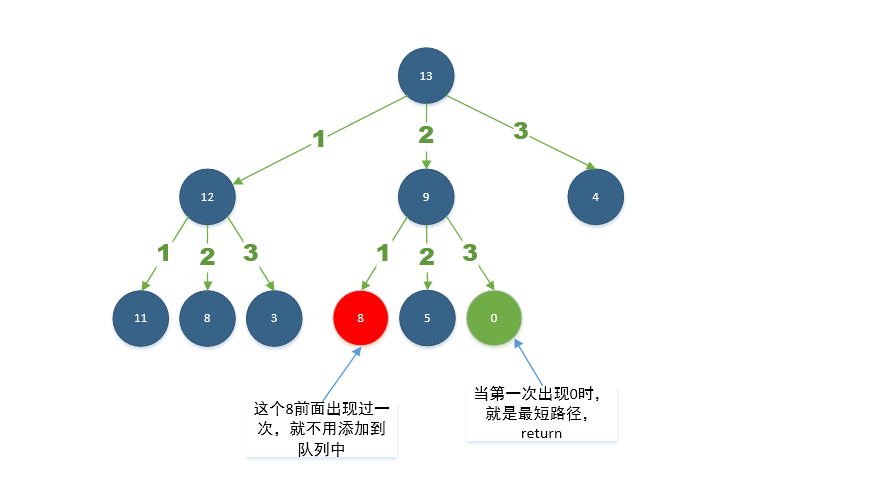# 队列和广度优先搜索## 模版

### 标准模版

int BFS(Node root, Node target) {
Queue<Node> queue = new LinkedList<>();  // 存储所有等待处理的节点
int step = 0;       // 深度
// 初始化，将根节点存入队列
queue.offer(root);
// BFS
while (!queue.isEmpty()) {
step = step + 1;
// 迭代
int size = queue.size();
for (int i = 0; i < size; ++i) {
Node cur = queue.peek();
// 第一次找到该节点，将深度返回
if (cur == target)
return step
for (Node next : the neighbors of cur) {
queue.offer(next);
}
queue.remove();
}
}
return -1;
}


1.如代码所示，在每一轮中，队列中的结点是等待处理的结点。
2.在每个更外一层的 while 循环之后，我们距离根结点更远一步。变量 step 指示从根结点到我们正在访问的当前结点的距离。

### 避免重复访问节点

int BFS(Node root, Node target) {
Queue<Node> queue = new LinkedList<>();  // 存储所有等待处理的节点
Set<Node> used = new HashSet<>();   // 存储已经访问了的节点
int step = 0;       // 深度

// 初始化，将根节点存入队列和 HashSet 中
queue.offer(root);

// BFS
while (!queue.isEmpty()) {
step = step + 1;
// 迭代
int size = queue.size();
for (int i = 0; i < size; ++i) {
Node cur = queue.peek();
// 第一次找到该节点，将深度返回
if (cur == target)
return step
for (Node next : the neighbors of cur) {
if(!used.contains(next)) {
queue.offer(next);
}
}
queue.remove();
}
}
return -1;
}


1.你完全确定没有循环，例如，在树遍历中；
2.你确实希望多次将结点添加到队列中。

## 例题

### 1.岛屿数量

• 难度：Medium

11110
11010
11000
00000

11000
11000
00100
00011

#### 解题思路及实现

class Solution {
public int numIslands(char[][] grid) {
int result = 0;
if (grid == null || grid.length == 0 || grid.length == 0) return result;

int nr = grid.length;
int nc = grid.length;

for (int c = 0; c < nc; c++) {
for (int r = 0; r < nr; r++) {
// 如果是岛，返回结果加1，并将默认的坐标点标记为0
if (isIsland(grid, c, r)) {
result++;
makeVisited(grid, c, r);
// 开始广度优先搜索算法
while (!queue.isEmpty()) {
final int removed = queue.remove();
final int removedCol = removed % nc;
final int removedRow = removed / nc;
//         top
//  left  removed  right
//        bottom

// top
final int removeTop = removedRow - 1;
if (removeTop >= 0 && isIsland(grid, removedCol, removeTop)) {
makeVisited(grid, removedCol, removeTop);
}

// left
final int removeLeft = removedCol - 1;
if (removeLeft >= 0 && isIsland(grid, removeLeft, removedRow)) {
makeVisited(grid, removeLeft, removedRow);
}

// bottom
final int removeBottom = removedRow + 1;
if (removeBottom <= nr - 1 && isIsland(grid, removedCol, removeBottom)) {
makeVisited(grid, removedCol, removeBottom);
}

// right
final int removeRight = removedCol + 1;
if (removeRight <= nc - 1 && isIsland(grid, removeRight, removedRow)) {
makeVisited(grid, removeRight, removedRow);
}
}
}
}
}
return result;
}

// 返回二维数组对应的位置是否为岛
private boolean isIsland(char[][] grid, int c, int r) {
return grid[r][c] == '1';
}

// 标记二维数组对应的位置非岛
private void makeVisited(char[][] grid, int c, int r) {
grid[r][c] = '0';
}
}


### 2.打开转盘锁

• 难度：Medium

#### 解题思路及实现

public class B752OpenLock {

public int openLock(String[] deadends, String target) {

// 用于bfs的队列

// 额外的一个哈希集，确保我们永远不会访问一个结点两次。
// 否则，我们可能陷入无限循环
Set<String> seen = new HashSet<>();
int depth = 0;

while (!queue.isEmpty()) {
final String node = queue.remove();

if (node == null) {
// null 作为单次广度搜索的尾巴标记
depth++;
if (queue.peek() != null) {
// 这意味着单轮搜索结束，队列末尾继续追加一个null
// 当轮训到下一个null时，意味着下轮的搜索结束
queue.offer(null);
}
} else if (node.equals(target)) {
return depth;
} else if (!dead.contains(node)) {  // 如果某个节点不是死亡数字
// 对于每一个状态，它可以扩展到最多 8 个状态，即将它的第 i = 0, 1, 2, 3 位增加 1 或减少 1
for (int i = 0; i < 4; i++) {           // 四个位置
for (int j = -1; j <= 1; j += 2) {  // 每个位置分别 -1 , + 1
// 这里用到一个小技巧避免对字符进行额外的负数判断
// 这里统一加10求余，值一定是期望的值，否则结果会返回负数
// 比如，当node.charAt(i) = '0'， j = -1 时，这时候结果仍然是期望的9，而不是 -1
int y = ((node.charAt(i) - '0') + j + 10) % 10;
// 取得对应的邻居节点，比如 i = 1, j = 1 时， '0000' -> '0100'
String nei = node.substring(0, i) + String.valueOf(y) + node.substring(i + 1);
if (!seen.contains(nei)) {
queue.offer(nei);
}
}
}
}
}
return -1;
}
}


### 3.完全平方数

• 难度：Medium

#### 解题思路及实现class Solution {
public int numSquares(int n) {
boolean[] visited = new boolean[n];
int step = 1;

while (!queue.isEmpty()) {
Integer removed = queue.remove();
// bfs
for (int i = 1; ; i++) {
if (removed == null) {
step++;
if (queue.peek() != null) {
queue.offer(null);
}
break;
}

int nextValue = removed - (i * i);

if (nextValue == 0)
return step;

if (nextValue < 0) {
break;
}

// 关键点！
// 当再次出现时没有必要加入，因为在该节点的路径长度肯定不小于第一次出现的路径长
if (!visited[nextValue]) {
visited[nextValue] = true;
}
}
}
return -1;
}
}


## 参考 & 感谢

• https://leetcode-cn.com/explore/learn/card/queue-stack/217/queue-and-bfs/869/

• https://leetcode-cn.com/problems/number-of-islands
• https://leetcode-cn.com/problems/open-the-lock
• https://leetcode-cn.com/problems/perfect-squares

## 关于我

Hello，我是 却把清梅嗅 ，如果您觉得文章对您有价值，欢迎 ❤️，也欢迎关注我的 博客 或者 GitHub